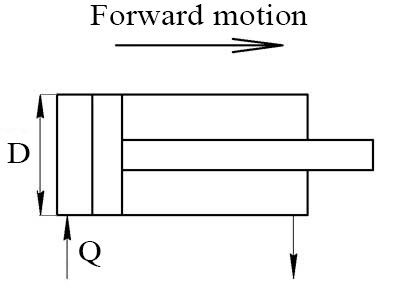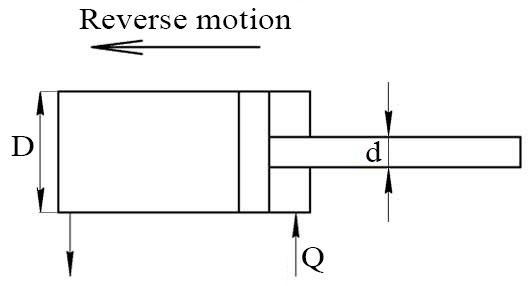# Hydraulic Cylinder Velocity Calculator

The velocity at which the piston rod of a hydraulic cylinder moves is based on the following equation:

V=Q/Ae
• V - velocity rate of piston rod
• Q - flow
• Ae - effective area

## Extended speed of cylinderOil flow Q: Units m3/s m3/min m3/h gpm lpm in3/min Piston diameter D: Units mm Millimetre cm Centimeter dm Decimeter m Meter km Kilometer in Inch

## Retracted speed of cylinderOil flow Q: Units m3/s m3/min m3/h gpm lpm in3/min Piston diameter D: Rod diameter d: Units mm Millimetre cm Centimeter dm Decimeter m Meter km Kilometer in Inch

Languages
Spanish (ES) Deutsch (DE)
Featured articles
How to read hydraulic circuits
Hydraulic cylinders
Hydraulic accumulators
Best calculators
Pressure loss calculator
Calculating the system curve
Hydraulic cylinder force calculator
My Youtube channel
This site uses cookies to store information on your computer. Details